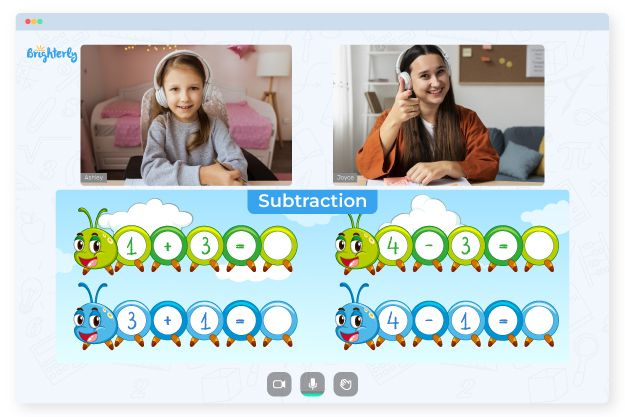# Number Line Subtraction Worksheets

Number line subtraction is a method for teaching and understanding subtraction with the help of a number line to represent the operation visually. To subtract on a number line, start at the minuend and move left the value of the number of units equal to the subtrahend. The final point on the number line represents the difference.

Practicing with the number line subtraction worksheet is an effective way to help kids learn to master subtraction. The worksheet provides a variety of practice questions to teach students to rapidly read and solve subtraction issues. Learning subtraction with the worksheet is essential in preparing kids for more challenging math concepts.

Math for Kids

Is Your Child Struggling With Math?
1:1 Online Math Tutoring## Benefits of subtraction on a number line worksheet

For second-graders, subtraction on the number line is essential to prepare them for tougher number line questions in subsequent levels. Considering the complexity of the grade, you ease learning by exposing kids to the open number line subtraction 2nd grade worksheet.

This worksheet teaches subtraction by moving left on the number line instead of right when adding. It also encourages students to learn using a visual-based approach to subtraction on a number line. The resources are designed with desirable everyday images and catchy colors to attract pupils’ attention.

As your students learn with the subtraction number line worksheets, you can rest assured that they will have a good time. Since the worksheets are interactive, your kids will be energized by learning.### Number Line Subtraction Worksheets PDF

Number Line Subtraction Worksheet### Number Line Subtraction Worksheets PDF

Open Number Line Subtraction 2nd Grade Worksheet### Number Line Subtraction Worksheets PDF

Subtraction Number Line Worksheets### Number Line Subtraction Worksheets PDF

Subtraction On A Number Line Worksheet

Struggling with Subtraction?• Does your child need extra help in understanding subtraction?
• An online tutor could help.

Is your child struggling to understand subtraction? Consider lessons with an online tutor.